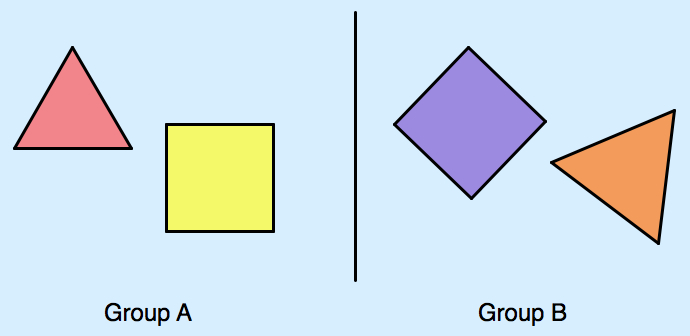# A Hidden Polygons Puzzle

Take a look at the two groups of shapes below. Both groups contain an equilateral triangle and a square. Now imagine that you showed students each group and asked them to identify the shapes. Do you think students would do equally well in naming the shapes in group A and group B?As you probably suspect, identifying the square and equilateral triangle in group B is harder. Research shows that students do a better job at naming geometric objects when they are oriented in an “upright” position with their bases horizontal to the page or the screen.

Part of the blame lies with textbooks. Students are more likely to see squares and equilateral triangles drawn upright than tilted at an angle. How can we help students expand their visual definitions of an equilateral triangle and a square so that they are better able to recognize them?

In the interactive Sketchpad game below, the goal is to spot an equilateral triangle and a square. There are eight points scattered across the screen: Three of the points form the vertices of an equilateral triangle, four of the points form the vertices of a square, and the remaining point is extraneous, included solely as a distraction.

Drag the triangle and the quadrilateral onto the points so that the triangle is equilateral and the quadrilateral is square. Press Show Answer to check your solution and press New Puzzle to generate a new, random challenge.

As an adult, I find the game surprisingly fun to play and even, at times, challenging. But the real audience for this game is students. By identifying equilateral triangles and squares in a variety of orientations, will students become more adept at recognizing the shapes? Try this game with your students and let me know how they fare!

An annotated list of all our elementary-themed blog posts is here.